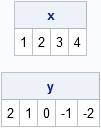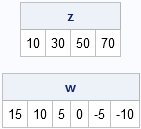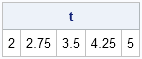It is often useful to create a vector with elements that follow an arithmetic sequence. For example, {1, 2, 3, 4} and {10, 30, 50, 70} are vectors with evenly spaced values. This post describes several ways to create vectors such as these.

The SAS/IML language has two ways to generate vectors with evenly spaced values: the colon operator (which SAS/IML documentation calls the "index creation operator") and the DO function.

### The Colon Operator

The colon operator enables you to create a sequence of values that differ by 1 or -1. The syntax is

x = first : last;
as shown in the following examples:

```proc iml; x = 1:4; /** increasing sequence **/ y = 2:-2; /** decreasing sequence **/ print x, y;```Notice that you get a decreasing sequence of numbers if the first parameter is less than the last parameter.

### The DO Function

Use the DO function when the increment between adjacent values is not 1 or -1. The syntax is

z = do(first, last, increment);
as shown in the following examples:

```z = do(10, 70, 20); /** positive increment **/ w = do(15, -10, -5); /** negative increment **/ print z, w;```### Linearly Spaced Vectors

Sometimes it is convenient to generate a vector of n evenly spaced points between (and including) two values a and b. To do this, use an interval of length (b-a)/(n-1). (Notice that you divide by n – 1 because there are n – 1 intervals in a sequence that contains n points.) If you generate these sequences often, you can define a module to encapsulate the task:

```/** generate n evenly spaced points between (and including) a and b **/ start Linspace(a, b, n); if n<2 then return( b ); incr = (b-a) / (n-1); return( do(a, b, incr) ); finish;   t = Linspace(2, 5, 5); print t;```More information about creating vectors with certain properties is contained in the "Getting Started" chapter of my book Statistical Programming with SAS/IML Software, which you can download from my SAS Press author page.

Share